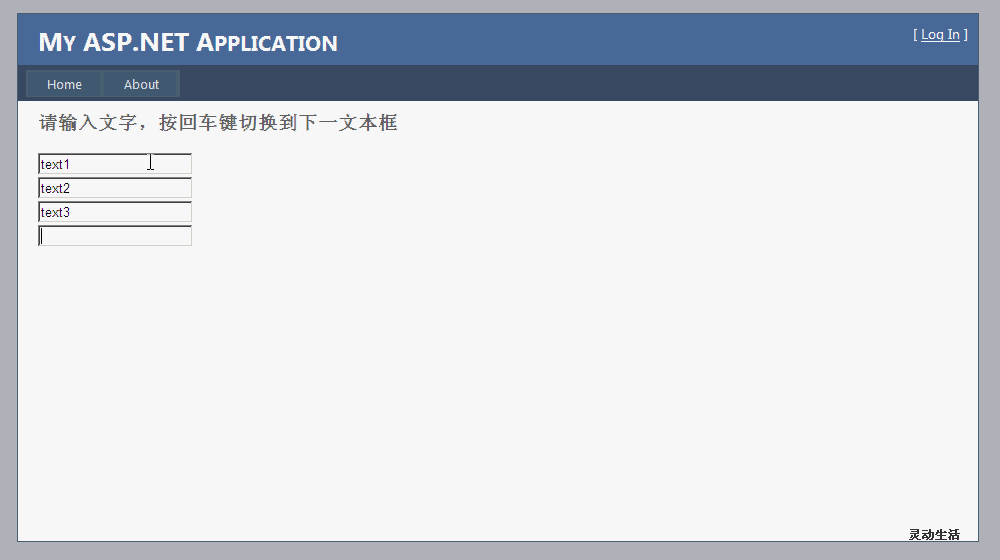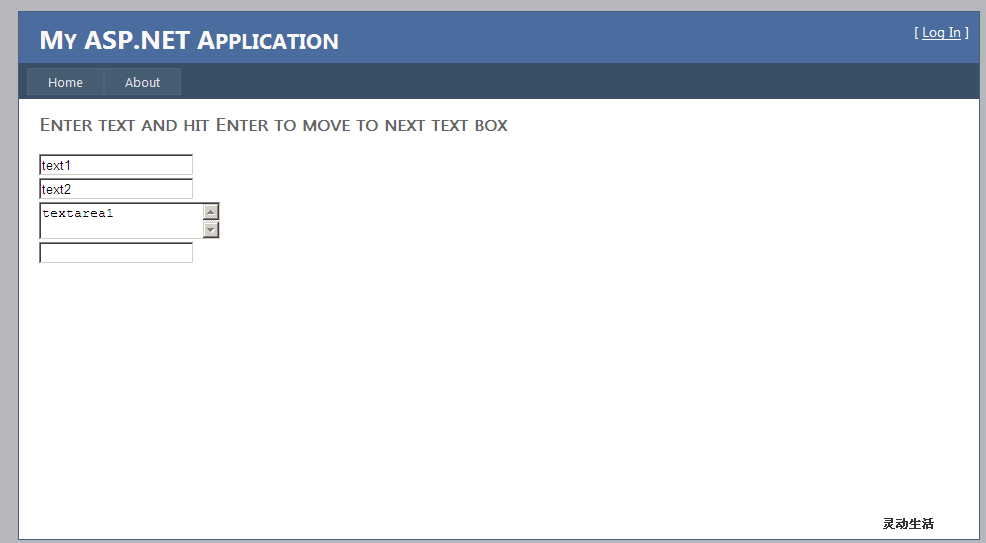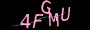×

# 基于Jquery的实现回车键Enter切换焦点

1、 首先引用Jquery类库
<script src="Scripts/jquery-1.4.1.min.js" type="text/javascript"></script>
2、 Javascript代码

<script type="text/javascript">
\$(function () {
\$('input:text:first').focus();
var \$inp = \$('input:text');
\$inp.bind('keydown', function (e) {
var key = e.which;
if (key == 13) {
e.preventDefault();
var nxtIdx = \$inp.index(this) + 1;
\$(":input:text:eq(" + nxtIdx + ")").focus();
}
});
});
</script>

\$('input:text:first').focus();

var \$inp = \$('input:text');

\$inp.bind('keydown', function (e) {}

var key = e.which;

e.preventDefault();

var nxtIdx = \$inp.index(this) + 1;

\$(":input:text:eq(" + nxtIdx + ")").focus();

3.、HTML代码

<div>
<asp:TextBox ID="txt1" runat="server" /><br />
<asp:TextBox ID="txt2" runat="server" /><br />
<asp:TextBox ID="txt3" runat="server" /><br />
<asp:TextBox ID="txt4" runat="server" /><br />
</div>

3、 运行程序4、 HTML代码

<div>
<asp:TextBox ID="tb1" runat="server" class="cls" /><br />
<asp:TextBox ID="tb2" runat="server" class="cls" /><br />
<asp:TextBox ID="tb3" TextMode="MultiLine" runat="server" class="cls" /><br />
<asp:TextBox ID="tb4" runat="server" class="cls" /><br />
</div>

5、 Javascript代码

<script type="text/javascript">
\$(function () {
\$('input:text:first').focus();
var \$inp = \$('.cls');
\$inp.bind('keydown', function (e) {
var key = e.which;
if (key == 13) {
e.preventDefault();
var nxtIdx = \$inp.index(this) + 1;
\$(".cls:eq(" + nxtIdx + ")").focus();
}
});
});
</script>

var \$inp = \$('.cls');

6、 运行效果https://www.jiangweishan.com/article/svg1486310400964.html

### 网友评论文明上网理性发言已有0人参与